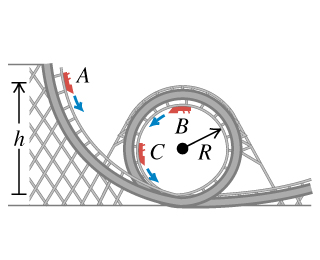# Problem: A car in an amusement park ride rolls without friction around a track . The car starts from rest at point A at a height h above the bottom of the loop. Treat the car as a particle.What is the minimum value of h (in terms of R) such that the car moves around the loop without falling off at the top (point B)?If the car starts at height h= 4.10 R and the radius is exttip{R_{ m 1}}{R_1} = 15.0 m , compute the speed of the passengers when the car is at point C, which is at the end of a horizontal diameter.Compute the radial acceleration of the passengers when the car is at point C, which is at the end of a horizontal diameter.Compute the tangential acceleration of the passengers when the car is at point C, which is at the end of a horizontal diameter.

⚠️Our tutors found the solution shown to be helpful for the problem you're searching for. We don't have the exact solution yet.

###### Problem Details

A car in an amusement park ride rolls without friction around a track. The car starts from rest at point A at a height h above the bottom of the loop. Treat the car as a particle.

What is the minimum value of h (in terms of R) such that the car moves around the loop without falling off at the top (point B)?

If the car starts at height h= 4.10 R and the radius is = 15.0 m , compute the speed of the passengers when the car is at point C, which is at the end of a horizontal diameter.

Compute the radial acceleration of the passengers when the car is at point C, which is at the end of a horizontal diameter.

Compute the tangential acceleration of the passengers when the car is at point C, which is at the end of a horizontal diameter.